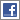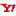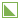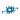# Costs & Delivery

## Calculating U-Values

Each component of your wall contributes to its overall thermal efficiency, commonly referred to as its 'R' value ('U' value).

The R-Value expressed as m²*K/W is the coefficient of thermal transmittance.

This is the amount of energy passing through a material or structure in one hour expressed in watts per square metre for a temperature difference between the two surfaces of 1°C (=1°Kelvin).

Hence, the higher the R value (the lower the U value), the better the insulation.

## How to calculate

Provided you know the thickness and thermal conductivity or Lambda (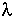) value and the μ = vapor diffusion resistance of each component, the R value and the dew point is quickly calculated using the formula:

(0,13 + 0,04 +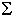( Width /))

Please enter your data in the fields below (the preset data is typical for a 250mm Styro Stone wall) and don't forget to complete the data for plaster, render etc. as well.

Theof Styro Stone's EPS (styrofoam) is 0.034. The vapour diffusion resistance depends on the density of our material, which is 25g/l.

 Width[m][W/mk] μ_min μ_max SD[m] R[m²K/W] T[°C] Dew point[°C] Innerrender/plaster Inner insulation wall structure(i.e.: concrete) Exterior insulation Exterior render

climate inhouse (°C): % rel. humidity

climate outside (°C): % rel. humidity

 bookmark at